# Euclidean Vectors in FlashThis post is part of a series called You Do The Math.
Playing Around with Elastic Collisions
Gravity in Action

Twice a month, we revisit some of our readers’ favorite posts from Activetuts+ history. This week’s retro-Active tutorial, first published in April, is a guide to Euclidean vectors: what they are, why you'd use them, and how to implement them in Flash with AS3.

Euclidean vectors are objects in geometry with certain properties that are very useful for developing games. They can be seen as points, but they also have a magnitude and a direction. They are represented as arrows going from the initial point to the final point, and that's how we will draw them in this article.

Euclidean vectors are commonly used in mathematics and physics for a lot of things: they can represent velocity, acceleration and forces in physics, or help prove a lot of important theorems in mathematics. In this tutorial, you'll learn about Euclidean vectors, and build a class that you can use in your own Flash projects.

Please note that Euclidean vectors are different than ActionScript's Vector class, and also different than vector drawing.

Vectors can be used in the Flash environment to help you achieve complex tasks that would otherwise require a lot of effort if done without them. In this article you will learn how to use them in Flash, as well as learn a lot of cool tricks with vectors.

## Step 1: Cartesian Coordinates and Flash's Coordinates

Before jumping into vectors, let's introduce Flash's coordinate system. You are probably familiar with the Cartesian coordinate system (even if you don't know it by name):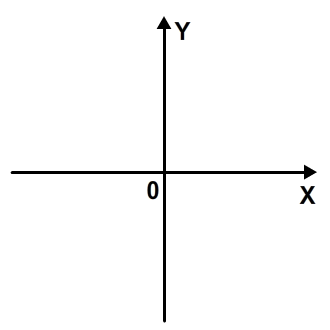Flash's system is very similar. The only difference is that the y-axis is upside-down: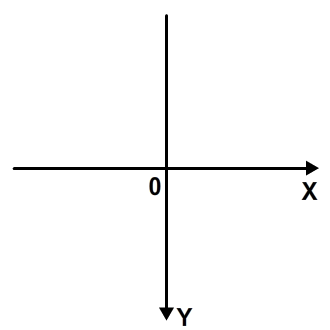When we start working with vectors in flash, we need to remember that. However, good news: this different system doesn't make much difference. Working with vectors in it will be basically like working with vectors in the Cartesian system.

## Step 2: Defining a Vector

For the purpose of this tutorial, we will define and work with all vectors' initial points as being the registration point of the stage, just as they are commonly used in mathematics. A vector will then be defined just like a common point, but it will have magnitude and angle properties. Take a look at some example vectors defined in the stage: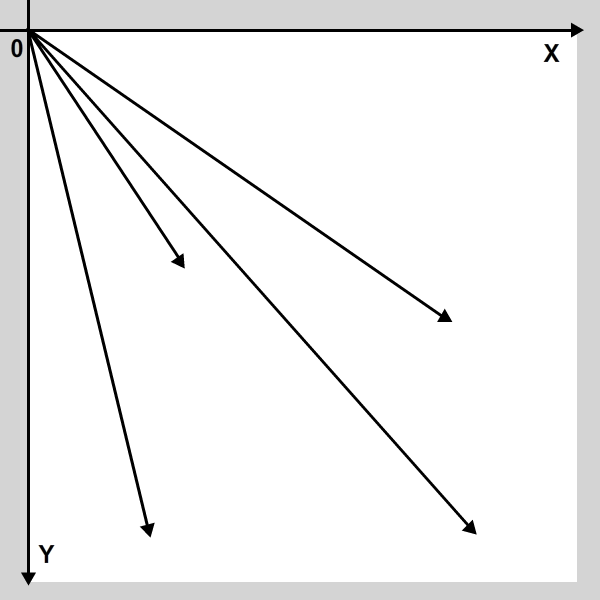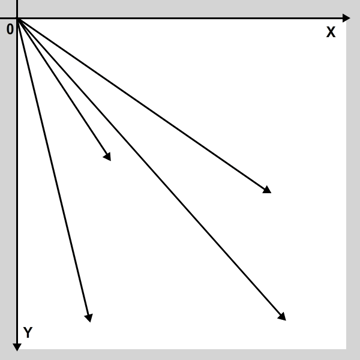As you can see, a vector is represented by an arrow, and each vector has a certain length (or magnitude) and points along a certain angle. The tail of each vector is at the registration point (0, 0).

We will create a simple EuclideanVector class for this tutorial, using the Point class to hold the vector's coordinates. Let's create the basic vector class now:

 1 2 package  3 {  4  import flash.geom.Point;  5   6  public class EuclideanVector  7  {  8  public var position:Point;  9  public var magnitude:Number;  10  public var angle:Number;  11   12  public function EuclideanVector(endPoint:Point)  13  {  14  position = endPoint;  15  }  16  }  17 } 

During this tutorial, we will talk about the sense and the direction of a vector. Note that the direction just defines a line that "contains" the vector. The sense is what defines which way the vector points along this line.

## Step 3: Inverse of a Vector

In this tutorial we will use the expression "inverse of a vector". The inverse of a vector is another vector with the same magnitude and direction, but a contrary sense. That translates to a vector with the opposite signal of the first vector's coordinates. So a vector with an endpoint of (x, y) would have an inverse vector with an endpoint of (-x, -y).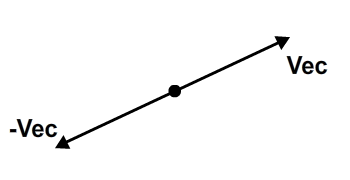Let's add a function to our EuclideanVector class to return the inverse vector:

 1 2 public function inverse():EuclideanVector  3 {  4  return new EuclideanVector(new Point(-position.x, -position.y));  5 } 

## Step 4: Basic Operations Addition

Now that we have learned how to define a vector, let's learn how to add two vectors: it's as simple as adding their coordinates separately. Look at this image: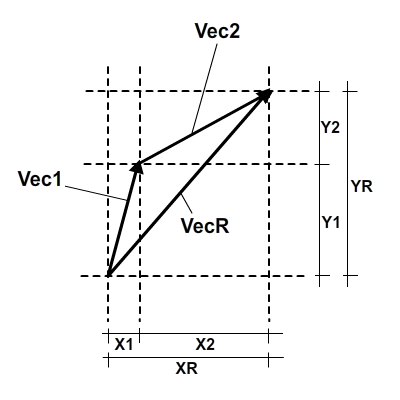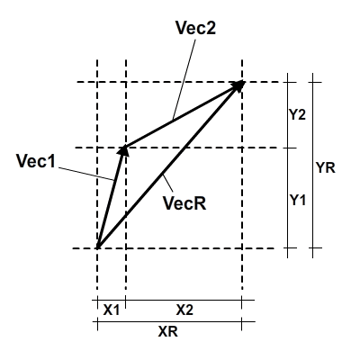If you notice in the image, the result of the addition of two vectors is another vector, and you can see that its coordinates are the sum of the coordinates of the other two vectors. In code, it would look like this:

 1 2 public function sum(otherVector:EuclideanVector):EuclideanVector  3 {  4  position.x += otherVector.position.x;  5  position.y += otherVector.position.y;  6   7  return this;  8 } 

So we can say that:

 1 2 vecR == vec1.sum(vec2); 

## Step 5: Basic Operations Subtraction

Subtraction works almost the same as addition, but instead we will be adding the inverse of the second vector to the first vector.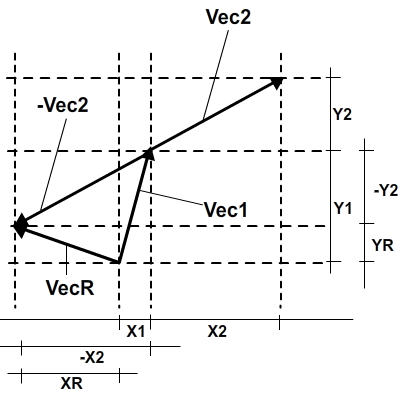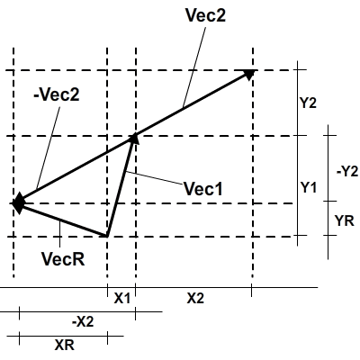It is already known how to sum two vectors, so here's the code for subtraction:

 1 2 public function subtract(otherVector:EuclideanVector):EuclideanVector  3 {  4  position.x -= otherVector.position.x;  5  position.y -= otherVector.position.y;  6   7  return this;  8 } 

This code is extremely useful to get a vector that goes from the point of a vector to the point of another. Look again at the image and you will see this is true. It will be used a lot in the later examples.

## Step 6: Basic Operations Multiplication by a Number

The multiplication between a vector and a number (regular numbers are known as "scalars" in vector math) results in a vector which has had magnitude multiplied by this number, but still pointing in the same direction; it's "stretched" if the scalar is larger than 1, and squashed if the scalar is between 0 and 1. The sense of the new vector will be the same as the original vector if the scalar is positive, or the opposite if negative. Basically, this number "scales" the vector. Look at the picture: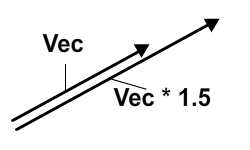In the code, we only multiply a vector's coordinates by the number, which will then scale the vector:

 1 2 public function multiply(number:Number):EuclideanVector  3 {  4  position.x *= number;  5  position.y *= number;  6   7  return this;  8 } 

## Step 7: Getting a Vector's Magnitude

In order to get a vector's magnitude, we will use the Pythagorean theorem. If you forgot what is it, here is a quick refresher: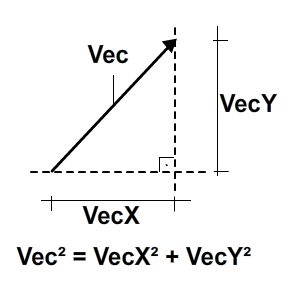The code is very simple:

 1 2 public function magnitude():Number  3 {  4  return Math.sqrt((position.x * position.x) + (position.y * position.y));  5 } 

You should also remove the line public var magnitude:Number, as this is what we'll use from now on.

The magnitude of a vector will always be positive, since it is the square root of the sum of two positive numbers.

## Step 8: Getting the Angle of a Vector

The angle of a vector is the angle between the x-axis and the vector's direction line. The angle is measured going from the x-axis and rotating anti-clockwise until the direction line in the cartesian system: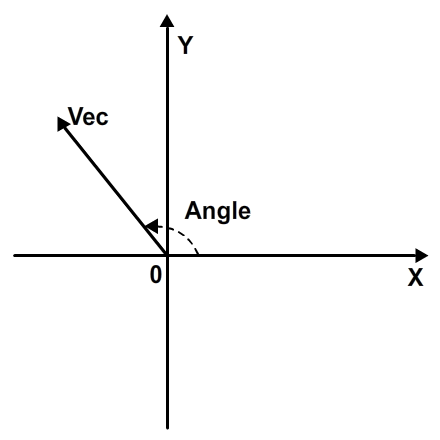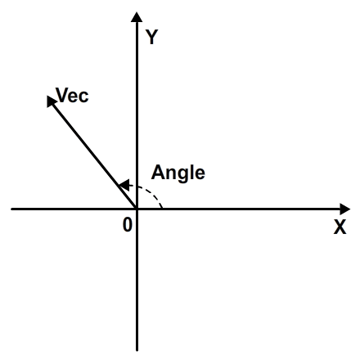However, in Flash's coordinate system, since the y-axis is upside down, this angle will be measured rotating clockwise: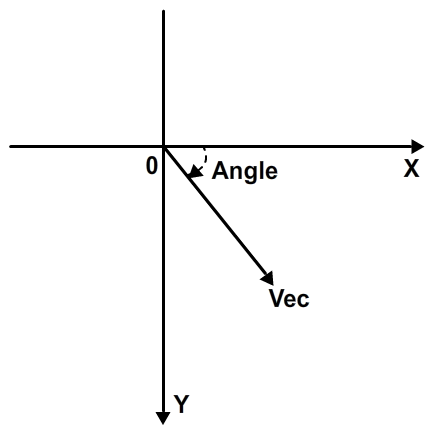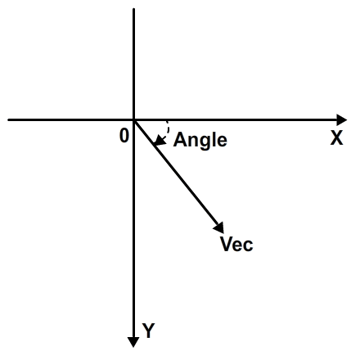This can be easily calculated using the following code. The angle will be returned in radians, in a range from 0 to 2pi. If you don't know what radians are or how to use them, this tutorial by Michael James Williams will help you a lot.

 1 2 public function angle():Number  3 {  4  var angle:Number = Math.atan2(position.y, position.x);  5   6  if (angle < 0)  7  {  8  angle += Math.PI * 2;  9  }  10   11  return angle;  12 } 

## Step 9: Dot Product

The dot product between two vectors is a number with apparently no meaning, but it has two useful uses. Let's first take a look at how the dot product can be calculated: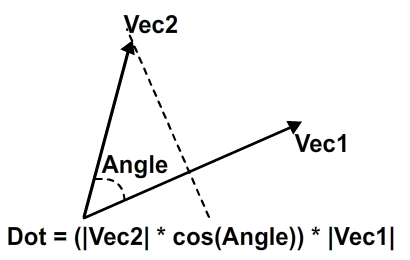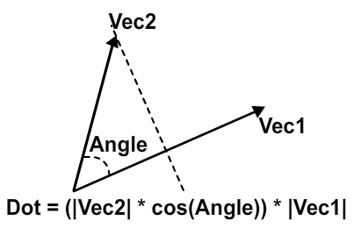But it also can be obtained by each vector's coordinates: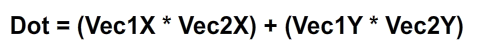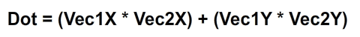The dot product can tell us a lot about the angle between the vectors: if it's positive, then the angle ranges from 0 to 90 degrees. If it's negative, the angle ranges from 90 to 180 degrees. If it's zero, the angle is 90 degrees. That happens because in the first formula only the cosine is responsible for giving the dot product a "signal": the magnitudes are always positive. But we know that a positive cosine means that the angle ranges from 0 to 90 degrees, and so on for negative cosines and zero.

The dot product can also be used to represent the length of a vector in the direction of the other vector. Think of it as a projection. This proves extremelly useful in things like the Separation of Axis Theorem (SAT) and its implementation in AS3 for collision detection and response in games.

Here is the practical code to get the dot product between two vectors:

 1 2 public function dot(otherVector:EuclideanVector):Number  3 {  4  return (position.x * otherVector.position.x) + (position.y * otherVector.position.y);  5 } 

## Step 10: Smallest Angle Between Vectors

The angle between vectors, as seen in Step 9, can be given by the dot product. Here is how to calculate it:

 1 2 public function angleBetween(otherVector:EuclideanVector):Number  3 {  4  return Math.acos(dot(otherVector) / (magnitude() * otherVector.magnitude()));  5 } 

## Step 11: Ranged Angle Between Vectors

There is also another way to calculate the angle, which gives results between -pi and pi and always calculates the angle that goes from the first vector to the second vector; this is useful when you want to easily integrate with a display object's rotation (which ranges from -180 to 180).

The method works by getting the angle for both vectors, then subtracting the angles and working on the result.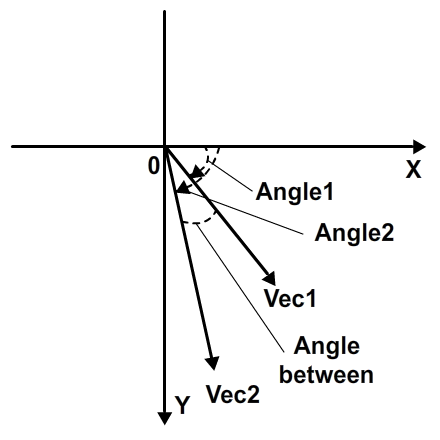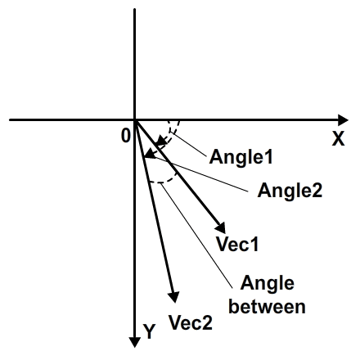The code:

 1 2 public function rangedAngleBetween(otherVector:EuclideanVector):Number  3 {  4  var firstAngle:Number;  5  var secondAngle:Number;  6   7  var angle:Number;  8   9  firstAngle = Math.atan2(otherVector.position.y, otherVector.position.x);  10  secondAngle = Math.atan2(position.y, position.x);  11   12  angle = secondAngle - firstAngle;  13   14  while (angle > Math.PI)  15  angle -= Math.PI * 2;  16  while (angle < -Math.PI)  17  angle += Math.PI * 2;  18   19  return angle;  20 } 

Note that this angle returns positive if secondAngle is higher than firstAngle, so the order in which you get the ranged angle will affect the result!

## Step 12: Normalizing a Vector

Normalizing a vector means making its magnitude be equal to 1, while still preserving the direction and sense of the vector. In order to do that, we multiply the vector by 1/magnitude. That way, its magnitude will be reduced, or increased, to 1.

 1 2 public function normalize():EuclideanVector  3 {  4  var m:Number = magnitude();  5  position.x /= m;  6  position.y /= m;  7   8  return this;  9 } 

## Step 13: Getting the Normal of a Vector

The normal of a vector is another vector that makes a 90 degree angle to the first. It can be calculated by the following formulas: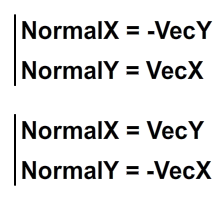The formulas rely on the fact that, since the normal is always perpendicular to a vector, we only need to change the order of the x and y coordinates and invert one of them in order to get a normal. The following image shows the process: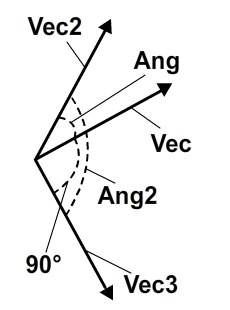In the image, Vec is the original vector, Vec2 is the vector with Vec's swapped coordinates, and Vec3 is a vector with Vec2's negative y coordinate. Ang and Ang2 are variable, but the angle between Vec and Vec3 is always 90 degrees.

And the code is simple

 1 2 public function normalRight():EuclideanVector  3 {  4  return new EuclideanVector(new Point(-position.y, position.x));  5 }  6 7 public function normalLeft():EuclideanVector  8 {  9  return new EuclideanVector(new Point(position.y, -position.x));  10 } 

## Step 14: Rotating a Vector

In order to rotate a vector, we assume the (0, 0) position (its initial point) will be the rotation center. The rotated point is given by the formula: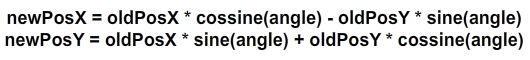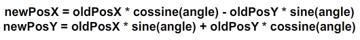This formula is obtained by applying a rotation matrix to that vector. We would be going beyond the scope of this tutorial if we went into the matrix and how it works, so I will just leave the formula here.

The code is pretty much the same:

 1 2 public function rotate(angleInRadians:Number):EuclideanVector  3 {  4  var newPosX:Number = (position.x * Math.cos(angleInRadians)) - (position.y * Math.sin(angleInRadians));  5  var newPosY:Number = (position.x * Math.sin(angleInRadians)) + (position.y * Math.cos(angleInRadians));  6   7  position.x = newPosX;  8  position.y = newPosY;  9   10  return this;  11 } 

This is the end of our basic vector operations. What you will see next is ways to use this class to do interesting things. Here is our class so far:

 1 2 package  3 {  4  import flash.geom.Point;  5   6  public class EuclideanVector  7  {  8  public var position:Point;  9  public var angle:Number;  10   11  public function EuclideanVector(endPoint:Point)  12  {  13  position = endPoint;  14  }  15   16  public function inverse():EuclideanVector  17  {  18  return new EuclideanVector(new Point(-position.x, -position.y));  19  }  20   21  public function sum(otherVector:EuclideanVector):EuclideanVector  22  {  23  position.x += otherVector.position.x;  24  position.y += otherVector.position.y;  25   26  return this;  27  }  28   29  public function subtract(otherVector:EuclideanVector):EuclideanVector  30  {  31  position.x -= otherVector.position.x;  32  position.y -= otherVector.position.y;  33   34  return this;  35  }  36   37  public function multiply(number:Number):EuclideanVector  38  {  39  position.x *= number;  40  position.y *= number;  41   42  return this;  43  }  44   45  public function magnitude():Number  46  {  47  return Math.sqrt((position.x * position.x) + (position.y * position.y));  48  }  49   50  public function angle():Number  51  {  52  var angle:Number = Math.atan2(position.y, position.x);  53   54  if (angle < 0)  55  {  56  angle += Math.PI * 2;  57  }  58   59  return angle;  60  }  61   62  public function dot(otherVector:EuclideanVector):Number  63  {  64  return (position.x * otherVector.position.x) + (position.y * otherVector.position.y);  65  }  66   67  public function angleBetween(otherVector:EuclideanVector):Number  68  {  69  return Math.acos(dot(otherVector) / (magnitude() * otherVector.magnitude()));  70  }  71   72  public function rangedAngleBetween(otherVector:EuclideanVector):Number  73  {  74  var firstAngle:Number;  75  var secondAngle:Number;  76   77  var angle:Number;  78   79  firstAngle = Math.atan2(otherVector.position.y, otherVector.position.x);  80  secondAngle = Math.atan2(position.y, position.x);  81   82  angle = secondAngle - firstAngle;  83   84  while (angle > Math.PI)  85  angle -= Math.PI * 2;  86  while (angle < -Math.PI)  87  angle += Math.PI * 2;  88   89  return angle;  90  }  91   92  public function normalize():EuclideanVector  93  {  94  position.x /= magnitude();  95  position.y /= magnitude();  96   97  return this;  98  }  99   100  public function normalRight():EuclideanVector  101  {  102  return new EuclideanVector(new Point(-position.y, position.x));  103  }  104   105  public function normalLeft():EuclideanVector  106  {  107  return new EuclideanVector(new Point(position.y, -position.x));  108  }  109   110  public function rotate(angleInRadians:Number):EuclideanVector  111  {  112  var newPosX:Number = (position.x * Math.cos(angleInRadians)) - (position.y * Math.sin(angleInRadians));  113  var newPosY:Number = (position.x * Math.sin(angleInRadians)) + (position.y * Math.cos(angleInRadians));  114   115  position.x = newPosX;  116  position.y = newPosY;  117   118  return this;  119  }  120  }  121 } 

## Step 15: Determining Whether a Point is Inside a Polygon

The action begins here. Determining whether a point lies inside a polygon or not is a very interesting topic, and there are many methods of achieving it. In this article I will present the three methods that are generally used:

• The crossing number or even-odd rule algorithm, which determines whether a point is inside a polygon from the number of edges that a "ray" cast from the point to infinity crosses.
• The winding number algorithm, which gives the answer based on the sum of all angles formed between consecutive vertices of a polygon and the point to check.
• The convex polygon algorithm, which, as the name says, only works for convex polygons and is based on whether or not a point is on a certain "side" of every edge of the polygon.

All these algorithms will rely on the fact that you know the coordinates of the vertices (corners) that define the polygon.

## Step 16: The Crossing Number or Even-Odd Rule Algorithm

This algorithm can be used for any shape. This is what you read: any shape, have it holes or not, be it convex or not. It is based on the fact that any ray cast from the point you want to check out to infinity will cross an even number of edges if the point is outside the shape, or odd number of edges if the point is inside the shape. This can be proven by the Jordan curve theorem, which implies that you will have to cross a border between some region and other region if you want to move from one to other. In our case, our regions are "inside the shape" and "outside the shape".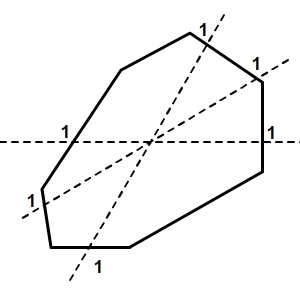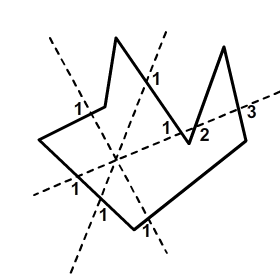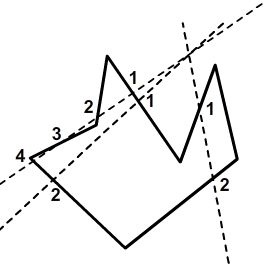The code for this algorithm is the following:

 1 2 public function isPointInsideShape1(point:EuclideanVector, shapeVertices:Vector.):Boolean  3 {  4  var numberOfSides:int = shapeVertices.length;  5   6  var i:int = 0;  7  var j:int = numberOfSides - 1;  8   9  var oddNodes:Boolean = false;  10   11  while (i < numberOfSides)  12  {  13  if ((shapeVertices[i].position.y < point.position.y && shapeVertices[j].position.y >= point.position.y) ||  14  (shapeVertices[j].position.y < point.position.y && shapeVertices[i].position.y >= point.position.y))  15  {  16  if (shapeVertices[i].position.x + (((point.position.y - shapeVertices[i].position.y) / (shapeVertices[j].position.y - shapeVertices[i].position.y)) *  17  (shapeVertices[j].position.x - shapeVertices[i].position.x)) < point.position.x)  18  {  19  oddNodes = !oddNodes;  20  }  21  }  22   23  j = i;  24   25  i++;  26  }  27   28  return oddNodes;  29 } 

It will return false if the point is not inside the shape, or true if the point is inside the shape.

## Step 17: The Winding Number Algorithm

The winding number algorithm use the sum of all the angles made between the point to check and each pair of points that define the polygon. If the sum is close to 2pi, then the point being checked is inside the vector. If it is close to 0 then the point is outside.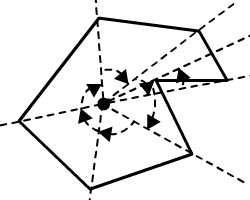1 2 public function isPointInsideShape2(point:EuclideanVector, shapeVertices:Vector.):Boolean  3 {  4  var numberOfSides:int = shapeVertices.length;  5   6  var i:int = 0;  7  var angle:Number = 0;  8   9  var rawAngle:Number = 0;  10   11  var firstVector:EuclideanVector;  12  var secondVector:EuclideanVector;  13   14  while(i < numberOfSides)  15  {  16  firstVector = new EuclideanVector(new Point(shapeVertices[i].position.x - point.position.x, shapeVertices[i].position.y - point.position.y));  17  secondVector = new EuclideanVector(new Point(shapeVertices[(i + 1) % numberOfSides].position.x - point.position.x, shapeVertices[(i + 1) % numberOfSides].position.y - point.position.y));  18   19  angle += secondVector.rangedAngleBetween(firstVector);  20   21  i++;  22  }  23   24  if(Math.abs(angle) < Math.PI)  25  return false;  26  else  27  return true;  28 } 

The code uses the ranged angle between vectors and gives space for imprecisions: notice how we are checking the results of the sum of all angles. We do not check if the angle is exactly zero or 2pi. Instead, we check if it is less than pi and higher than pi, a considerable median value.

## Step 18: The Concave Polygon Algorithm

The concave polygon algorithm relies on the fact that, for a concave polygon, a point inside it is always to the left of the edges (if we are looping through them in a counter-clockwise sense) or to the right of the edges (if we are looping through them in a clockwise sense).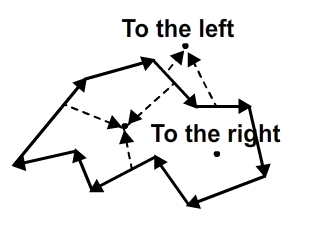Imagine standing in a room shaped like the image above, and walking around the edges of it with your left hand trailing along the wall. At the point along the wall where you are closest to the point you are interested in, if it's on your right then it must be inside the room; if it's on your left then it must be outside.

The problem lies in determining whether a point is to the left or right of an edge (which is basically a vector). This is done through the following formula: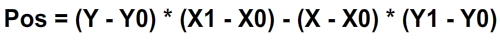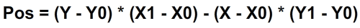That formula returns a number less than 0 for points to the right of the edge, and greater than 0 for points to the left of it. If the number is equal to 0, the point lies on the edge, and is considered inside the shape. The code is the following:

 1 2 public function isPointInsideShape3(point:EuclideanVector, shapeVertices:Vector.):Boolean  3 {  4  var numberOfSides:int = shapeVertices.length;  5   6  var i:int = 0;  7   8  var firstEdgePoint:EuclideanVector;  9  var secondEdgePoint:EuclideanVector;  10   11  var leftOrRightSide:Boolean;  12   13  while(i < numberOfSides)  14  {  15  firstEdgePoint = shapeVertices[i];  16  secondEdgePoint = shapeVertices[(i + 1) % numberOfSides];  17   18  if(i == 0)  19  {  20  // Determining if the point is to the left or to the right of first edge  21  // true for left, false for right  22   23  leftOrRightSide = ((point.position.y - firstEdgePoint.position.y) * (secondEdgePoint.position.x - firstEdgePoint.position.x)) - ((point.position.x - firstEdgePoint.position.x) * (secondEdgePoint.position.y - firstEdgePoint.position.y)) > 0;  24  }  25  else  26  {  27  // Now all edges must be on the same side  28   29  if(leftOrRightSide && ((point.position.y - firstEdgePoint.position.y) * (secondEdgePoint.position.x - firstEdgePoint.position.x)) - ((point.position.x - firstEdgePoint.position.x) * (secondEdgePoint.position.y - firstEdgePoint.position.y)) < 0)  30  {  31  // Not all edges are on the same side!  32   33  return false;  34  }  35  else if(!leftOrRightSide && ((point.position.y - firstEdgePoint.position.y) * (secondEdgePoint.position.x - firstEdgePoint.position.x)) - ((point.position.x - firstEdgePoint.position.x) * (secondEdgePoint.position.y - firstEdgePoint.position.y)) > 0)  36  {  37  // Not all edges are on the same side!  38   39  return false;  40  }  41  }  42   43  i++;  44  }  45   46  // We looped through all vertices and didn't detect different sides  47   48  return true;  49 } 

This code works regardless of whether you have the shape's vertices defined clockwise or counter-clockwise.

## Step 19: Ray Casting

Ray casting is a technique often used for collision detection and rendering. It consists of a ray that is cast from one point to another (or out to infinity). This ray is made of points or vectors, and generally stops when it hits an object or the edge of the screen. Similarly to the point-in-shape algorithms, there are many ways to cast rays, and we will see two of them in this post:

• The Bresenham's line algorithm, which is a very fast way to determine close points that would give an approximation of a line between them.
• The DDA (Digital Differential Analyzer) method, which is also used to create a line.

In the next two steps we will look into both methods. After that, we will see how to make our ray stop when it hits an object. This is very useful when you need to detect collision against fast moving objects.

## Step 20: The Bresenham's Line Algorithm

This algorithm is used very often in computer graphics, and depends on the convention that the line will always be created pointing to the right and downwards. (If a line has to be created to the up and left directions, everything is inverted later.) Let's go into the code:

 1 2 public function createLineBresenham(startVector:EuclideanVector, endVector:EuclideanVector):Vector.  3 {  4  var points:Vector. = new Vector.();  5   6  var steep:Boolean = Math.abs(endVector.position.y - startVector.position.y) > Math.abs(endVector.position.x - startVector.position.x);  7   8  var swapped:Boolean = false;  9   10  if (steep)  11  {  12  startVector = new EuclideanVector(new Point(startVector.position.y, startVector.position.x));  13  endVector = new EuclideanVector(new Point(endVector.position.y, endVector.position.x));  14  }  15   16  // Making the line go downward  17  if (startVector.position.x > endVector.position.x)  18  {  19  var temporary:Number = startVector.position.x;  20   21  startVector.position.x = endVector.position.x;  22   23  endVector.position.x = temporary;  24   25  temporary = startVector.position.y;  26   27  startVector.position.y = endVector.position.y  28   29  endVector.position.y = temporary;  30   31  swapped = true;  32  }  33   34  var deltaX:Number = endVector.position.x - startVector.position.x;  35  var deltaY:Number = Math.abs(endVector.position.y - startVector.position.y);  36   37  var error:Number = deltaX / 2;  38   39  var currentY:Number = startVector.position.y;  40   41  var step:int;  42   43  if (startVector.position.y < endVector.position.y)  44  {  45  step = 1;  46  }  47  else  48  {  49  step = -1;  50  }  51   52  var iterator:int = startVector.position.x;  53   54  while (iterator < endVector.position.x)  55  {  56  if (steep)  57  {  58  points.push(new EuclideanVector(new Point(currentY, iterator)));  59  }  60  else  61  {  62  points.push(new EuclideanVector(new Point(iterator, currentY)));  63  }  64   65  error -= deltaY;  66   67  if (error < 0)  68  {  69  currentY += step;  70  error += deltaX;  71  }  72   73  iterator++;  74  }  75   76  if (swapped)  77  {  78  points.reverse();  79  }  80   81  return points;  82 } 

The code will produce an AS3 Vector of Euclidean vectors that will make the line. With this Vector, we can later check for collisions.

## Step 21: The DDA Method

An implementation of the Digital Differential Analyzer is used to interpolate variables between two points. Unlike the Bresenham's line algorithm, this method will only create vectors in integer positions for simplicity. Here's the code:

 1 2 public function createLineDDA(startVector:EuclideanVector, endVector:EuclideanVector):Vector.  3 {  4  var points:Vector. = new Vector.();  5 6  var dx:Number;  7  var dy:Number;  8 9  var _x:Number = startPoint.position.x;  10  var _y:Number = startPoint.position.y;  11 12  var m:Number;  13 14  var i:int;  15 16  dx = endPoint.position.x - startPoint.position.x;  17  dy = endPoint.position.y - startPoint.position.y;  18 19  if (Math.abs(dx) >= Math.abs(dy))  20  m = Math.abs(dx);  21  else  22  m = Math.abs(dy);  23 24  points.push(new EuclideanVector(new Point(int(_x), int(_y))));  25 26  i = 1;  27 28  while (i <= m)  29  {  30  _x += dx / m;  31  _y += dy / m;  32   33  points.push(new EuclideanVector(new Point(int(_x), int(_y))));  34   35  i++;  36  }  37   38  return points;  39 } 

This code will also return an AS3 Vector of Euclidean vectors.

## Step 22: Checking for Collisions Using Rays

Checking collision via rays is very simple. Since a ray consists of many vectors, we will check for collisions between each vector and a shape, until one is detected or the end of the ray is reached. In the following code, shapeToCheck will be a shape just like the ones we have been using in Steps 13-16. Here's the code:

 1 2 public function checkRayCollision(ray:Vector., shape:Vector.):Boolean  3 {  4  var rayLength:int = ray.length;  5   6  var i:int = 0;  7   8  while(i < rayLength)  9  {  10  if(isPointInsideShape1(ray[i], shape))  11  {  12  return true;  13  }  14   15  i++;  16  }  17   18  return false;  19 } 

You can use any point-inside-shape function you feel comfortable with, but pay attention to the limitations of the last one!

## Conclusion

You're ready to start using this knowledge everywhere now! It will be useful many times, and will save you a lot of extra calculations when trying to do more complex things in Flash.